Function Repository Resource:

# FractionMod

Get a congruent integer for a given fraction and modulus

Contributed by: Ed Pegg Jr
 ResourceFunction["FractionMod"][a/b,n] returns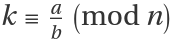such that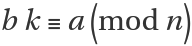.

## Details

Ifand GCD[b,n]=1 (i.e. b and n are coprime), then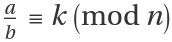.
ResourceFunction["FractionMod"] is frequently used in cryptography.

## Examples

### Basic Examples (2)

7×3 (mod 16) equals 5:

 In:=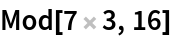Out=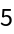Similarly, the fractional modulus of 3/5 (mod 16) equals 7:

 In:=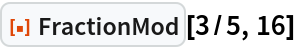Out=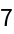As expected, adding 16 gives the same result (mod 16):

 In:=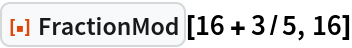Out=A grid of fractions, moduli and fractional moduli:

 In:=Out=### Scope (2)

A range of fractional moduli (mod 8) with tooltips:

 In:=Out=A few of the fractions and their fractional moduli (mod 8):

 In:=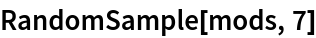Out=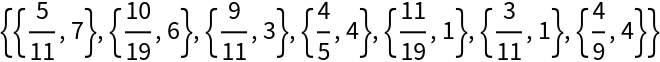### Neat Examples (1)

The fractional moduli of a series of prime denominator fractions gives a shuffling of the integer range:

 In:=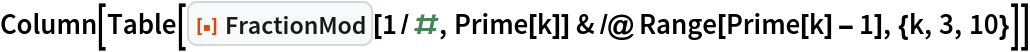Out=## Version History

• 1.0.0 – 11 October 2021